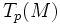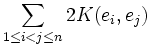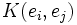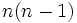# Scalar curvature

This article defines a notion of curvature for a differential manifold equipped with a Riemannian metric

This article defines a scalar function on a manifold, viz a function from the manifold to real numbers. The scalar function may be intrinsic or defined in terms of some other structure/functions

## Definition

Let$(M,g)$ be a Riemannian manifold.

### In terms of the Ricci curvature tensor

The scalar curvature associated to$(M,g)$ is defined as the trace of the Ricci curvature tensor of its Levi-Civita connection. By trace, we mean trace, when it is written as a symmetric bilinear form in terms of an orthonormal basis for the Riemannian metric.

### In terms of the Ricci curvature

The scalar curvature is a scalar function that associates a curvature at every point$p \in M$ as follows. Consider an orthonormal basis$e_i$ for$T_p(M)$. Then, the scalar curvature at$p$ is the sum of the Ricci curvatures for all vectors in the orthonormal basis.

### In terms of the sectional curvature

The scalar curvature at a point$p\in M$ is defined as follows. Let$e_i$ be an orthonormal basis at$p$. The scalar curvature is then:$\sum_{1 \le i < j \le n} 2K(e_i,e_j)$

where$K(e_i,e_j)$ denotes the sectional curvature of the plane spanned by$e_i$ and$e_j$.

### In terms of the Riemann curvature tensor

The scalar curvature can be viewed as a double-trace of the Riemann curvature tensor. A more explicit way of viewing it is as follows. Let$e_i$ be an orthonormal basis at$p$. The scalar curvature at$p$ is:$\sum_{1 \le i \le n, 1 \le j \le n} R(e_i,e_j,e_j,e_i)$

## Facts

### Scalar curvature in terms of Ricci curvature

If the manifold has dimension$n$, and if the Ricci curvature is constant at a point, the scalar curvature is$n$ times the Ricci curvature at that point.

If the sectional curvature is constant at the point, the scalar curvature is$n(n-1)$ times the sectional curvature.